Browse by Topic
Related Topics

# Mechanical Engineering Landing Gear Example: Wolfram SystemModeler

## Background

Explore the contents of this article with a free Wolfram SystemModeler trial. Teachers and textbook authors often need to simplify a real-world problem to pinpoint a specific area to work with—for instance, the examples in a textbook. However, even in real-world engineering, simplifying a problem can bring clarity when our understanding might otherwise drown in a sea of details. In this blog, we will design the landing gear for a helicopter. I have chosen the example of landing gear because the simplification to one degree of freedom gives accurate results and is typically how the problem is treated in textbooks. The solution is attainable through hand calculation. But a more subtle understanding of the problem can be gained using the Wolfram Language and Wolfram SystemModeler.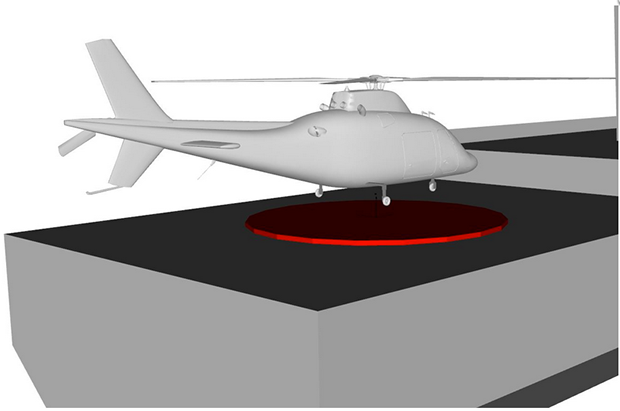First, let’s consider a real helicopter landing at sea in rough weather.

There are a few design criteria that need to be fulfilled during the landing. The numbers can vary a bit, but in this example we will use the helicopter AgustaWestland AW109.

A typical design specification for the landing gear is that the helicopter should be able to manage a free fall from 0.5 m. This is very similar to the max touchdown velocity of 10 feet/s, which also is a common spec.

The maximum allowable deflection for the landing gear is typically in the region 0.1–0.2 m. The reason for this is that the payload may be damaged otherwise. The task is to choose the stiffness and damping of the landing gear to minimize the peak landing force and deflection for a certain maximal acceleration during touchdown. The lower the peak force is, the lighter the landing gear can be.

The max takeoff weight is 2,850 kg; this is the weight we will use in this blog.

Both the AgustaWestland and a ship moving in waves have been modeled using the MultiBody library. An advanced model like this may be necessary in the design process; however, it is then hard to understand how all paramaters are coupled and to optimize, for instance, the landing gear. A simple landing on a steady ship is shown from different angles and in slow motion in the following video: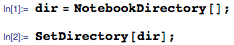The simplification of the helicopter drop test to a one-degree-of-freedom system would look like this: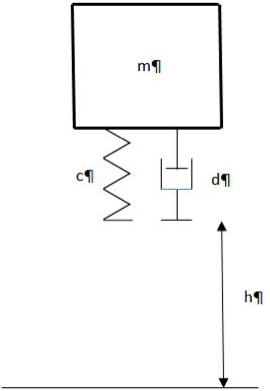A sketch at its equilibrium position (i.e. the spring has been compressed ystat due to gravity) can be seen below: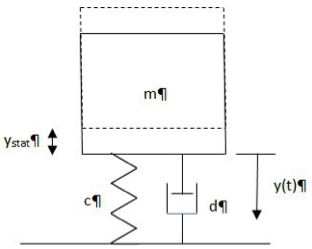Here, this system will be solved and analyzed both with the Wolfram Language and SystemModeler.

## Basic Equations

### Velocity before impact and maximum impact deflection

We start by determining the velocity just before impact, assuming the helicopter is dropped from height h=hdrop. We will use the following values: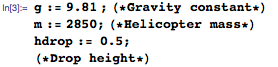We will also now introduce the optimal solution; this is just to plot relevant graphs during the example. The constants will be derived at the end: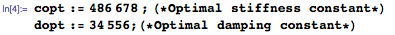The velocity of a mass dropped from height h will be: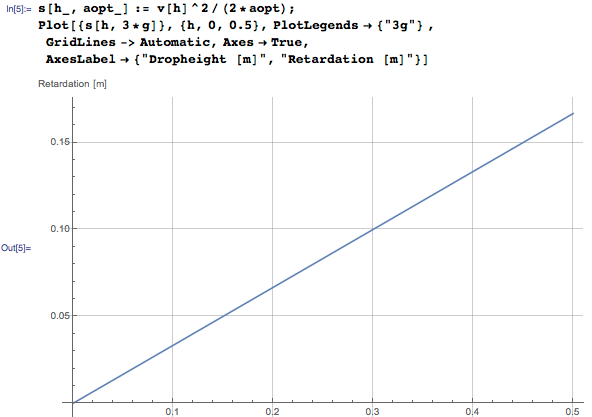We see that the absolute minimum deflection of the landing gear is 0.167 m if the helicopter is dropped from 0.5 m; the maximum deceleration should not exceed 3 g. That is, if we can design for constant deceleration, the deflection will otherwise be larger and/or the maximum force higher.

### Solving the one-degree-of-freedom system

With mass m [kg], damping constant d [Ns/m], and stiffness c [N/m], the equation of motion can be written as: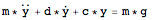For simplicity, in the Wolfram Language the equation will be rewritten as: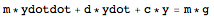The solution is found in almost all textbooks on mechanical vibrations. With the notation used here, it looks like the following: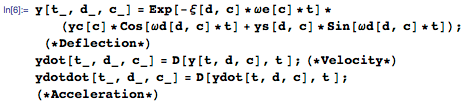The damping factor, natural frequency, and vibration frequency can be determined by: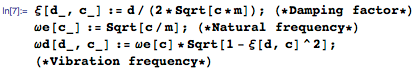Note that the origin of the coordinate system is in the equilibrium position.

There are two unknown constants, ys and yc, and we have two initial conditions—namely the position, which is the static deflection, and the velocity, which is the impact velocity:

y(t=0) =-m*g/c

ydot(t=0)=Sqrt[2*g*h]

The constants can be determined too: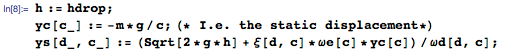The motion, velocity, and acceleration after impact may look like this: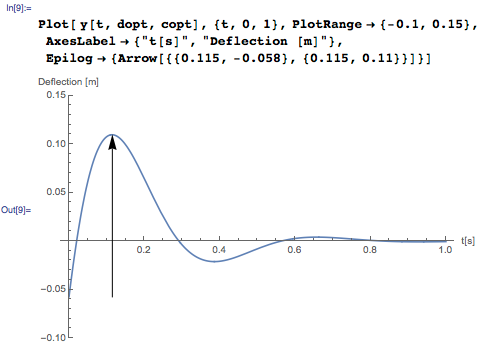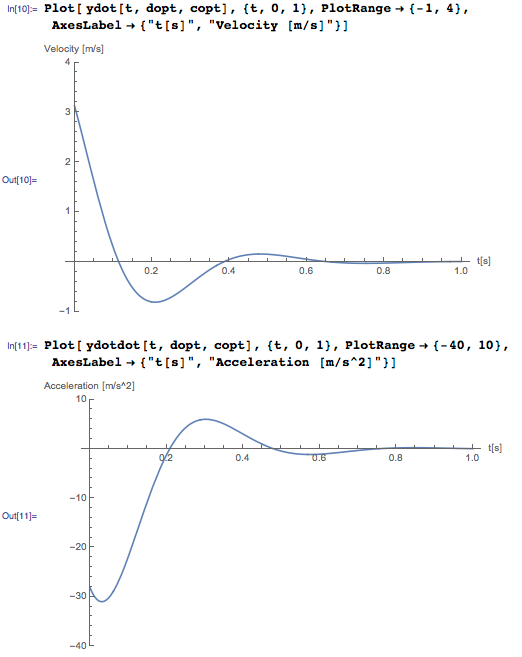From the above figures, it can be concluded that the helicopter will bounce back when the acceleration is zero, which occurs in this example at t=0.21 seconds. The speed will be ydot(0,21). If the deflection is less than -m*g/c after 0.21 seconds, the contact between the landing gear and the platform will stop. In this example, the absolute value of the deflection at approximately 0.38 seconds is less than at t=0. Consequently, there will be constant contact.

The mass, i.e. the helicopter, will bounce back after one period. It will leave at the same height as it hit the ground/landing platform: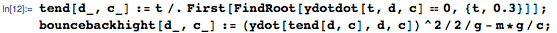We can see the effect of different stiffnesses and damping using the Wolfram Language Manipulate function. Note how the maximum deflection changes and also how the minimum values near t=0.38 seconds change. When the absolute value of this peak is larger than the static deflection (i.e. y), the contact will disappear. You can always get the initial settings for c and d back by clicking the plus sign in the top-left corner of the plot and choosing Initial Settings. Play around with the values in the notebook and try to understand why acceleration, forces, and displacements behave as they do: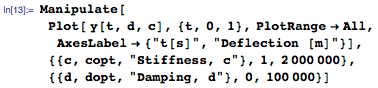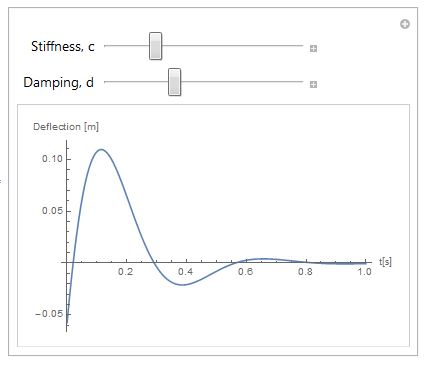Now the force on the landing gear and body is the deflection times the stiffness plus the velocity times the damping: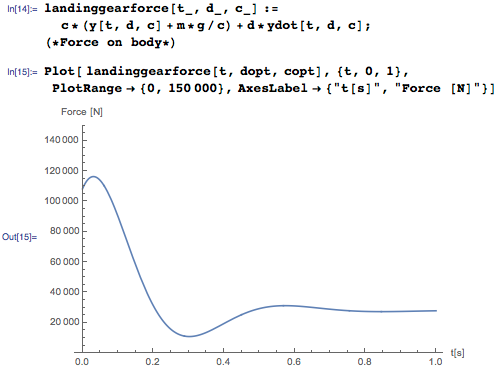### Optimize stiffness and damping for lowest force and acceleration

For illustration purposes, the smallest acceleration can be determined by: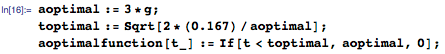Putting it all together, it is possible to investigate how different stiffnesses and damping will affect the bouncing, landing gear forces, and maximum acceleration of the components. No bouncing is allowed, and the force and acceleration will be minimized. Play around with the values in the notebook and see how the forces, bouncing, etc. change. The optimum values are the initial settings: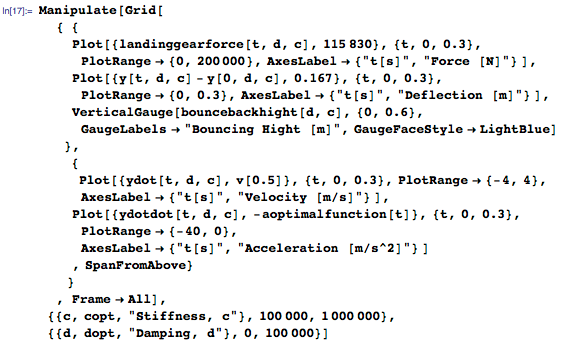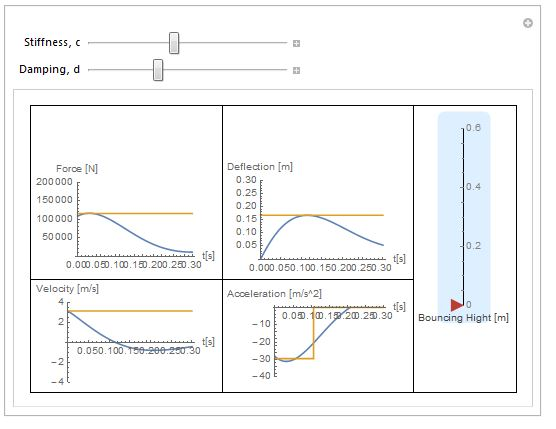## Simulation in Wolfram SystemModeler

The one-degree-of-freedom system is easy to build in SystemModeler. First, start WSMLink: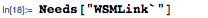Then import the file. Note that the complete path is needed. Another option is to first load the model in SystemModeler and then run this notebook: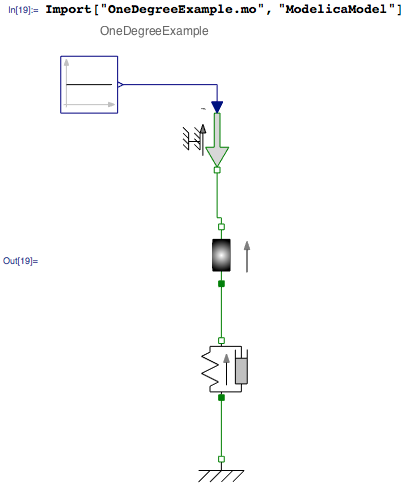Initialize and set parameter values: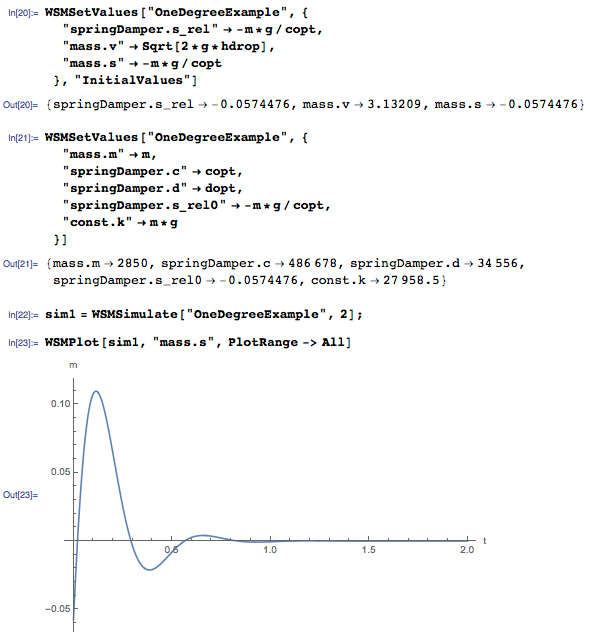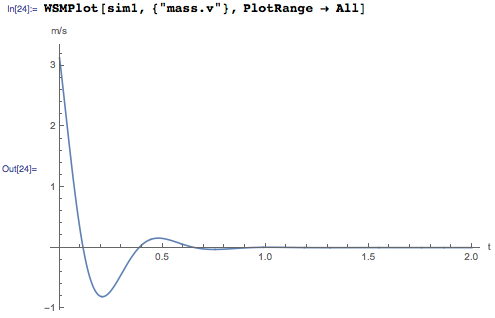Plot the results—for instance, the contact force: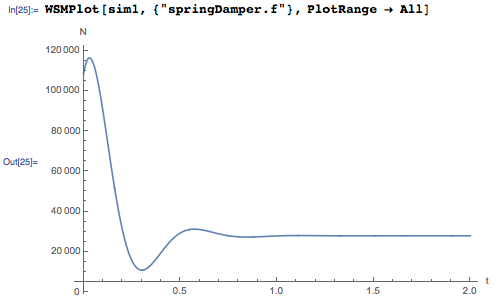## Optimization

### Optimizing the analytical solution

Although the landing gear has been simplified to a one-degree-of-freedom system, it is still not easy to find the optimum solution. Below is one example of how that can be done in the Wolfram Language. There are a few others, but this is one of the fastest: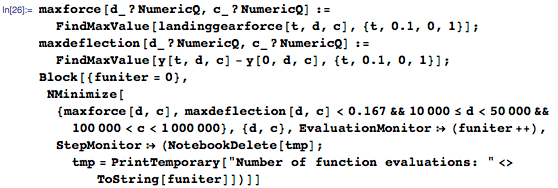### Optimizing using Wolfram SystemModeler

There are many other ways to do the same kind of optimization with results from SystemModeler. Here, I will show a way to run a parameter study and show the results in plots for visual inspection and understanding: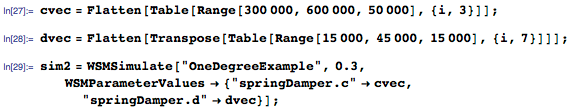First, plot all motions in the same plot: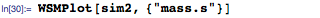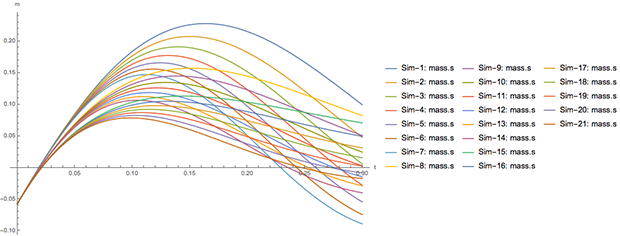Then in different plots: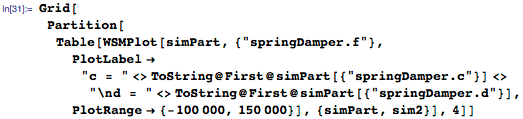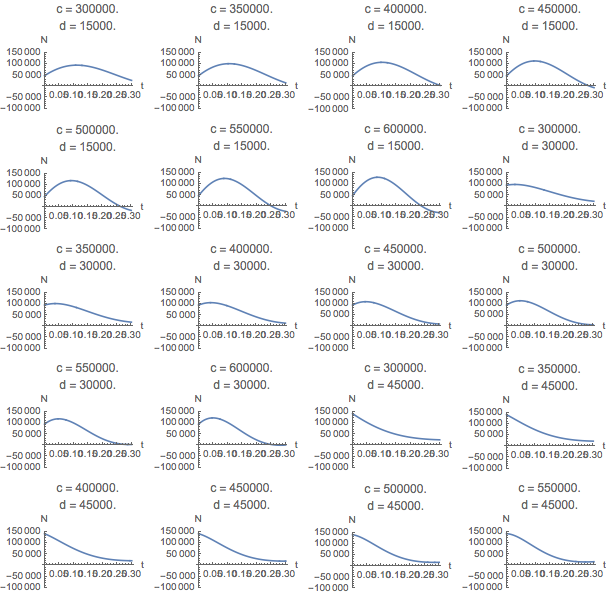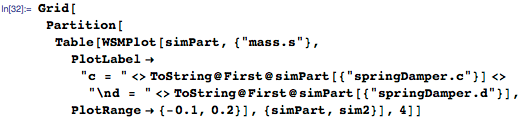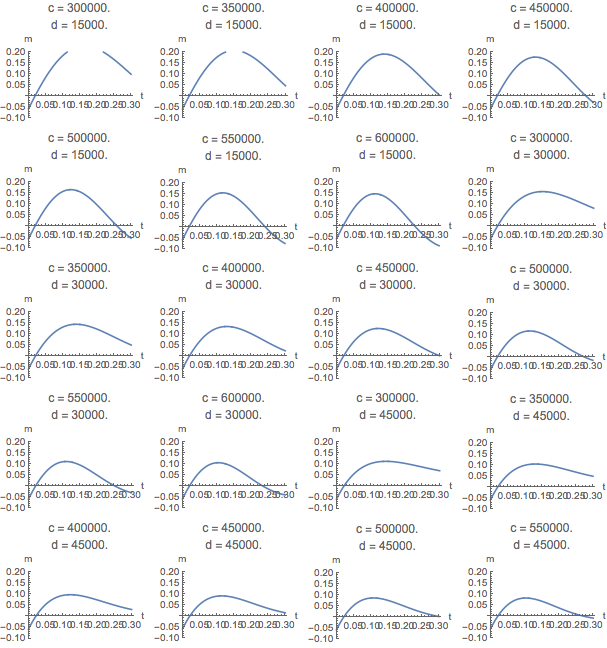We arrive at the same conclusion as with the analytical solution. The best solution is a spring-damper system 486,678 N/m and 34,556 Ns/m, although the exact numbers cannot be determined from the figures with the current step increments of damping and stiffness constants.

## Summary

We have seen that a task common in basic mechanical courses that has a straightforward solution can be time-consuming to solve if the parameters not are given. However, with the ability to easily vary parameters or questions, a better understanding is achieved. We used both the Wolfram Language and SystemModeler in this example. It is easy to see that if we increase complexity of the system to two degrees of freedom, hand calculation becomes too hard. Using SystemModeler, the solution will take just a little longer.

Further, we might reflect on how a rally car or motocross bike have been optimized: what are the costs in performance for a conventional car if optimized for a drop? The damping coefficient ξ became approximately 0.46 in the landing gear example. What is it for a modern car? Why the difference with the optimum here?

What was the spring doing? What was the damper doing? An increase in the damping coefficient can both increase and reduce the load on the mass.